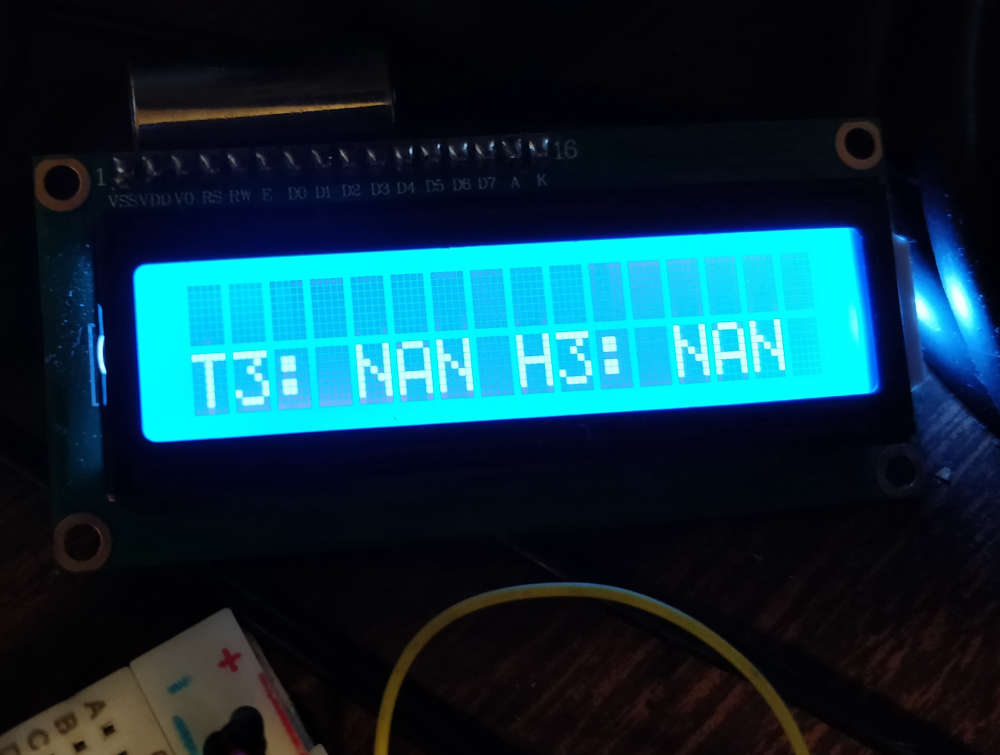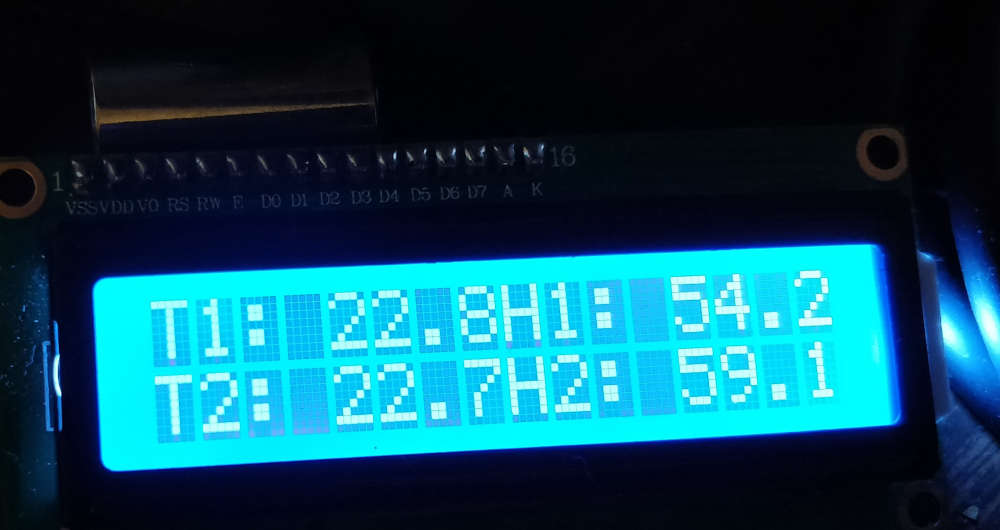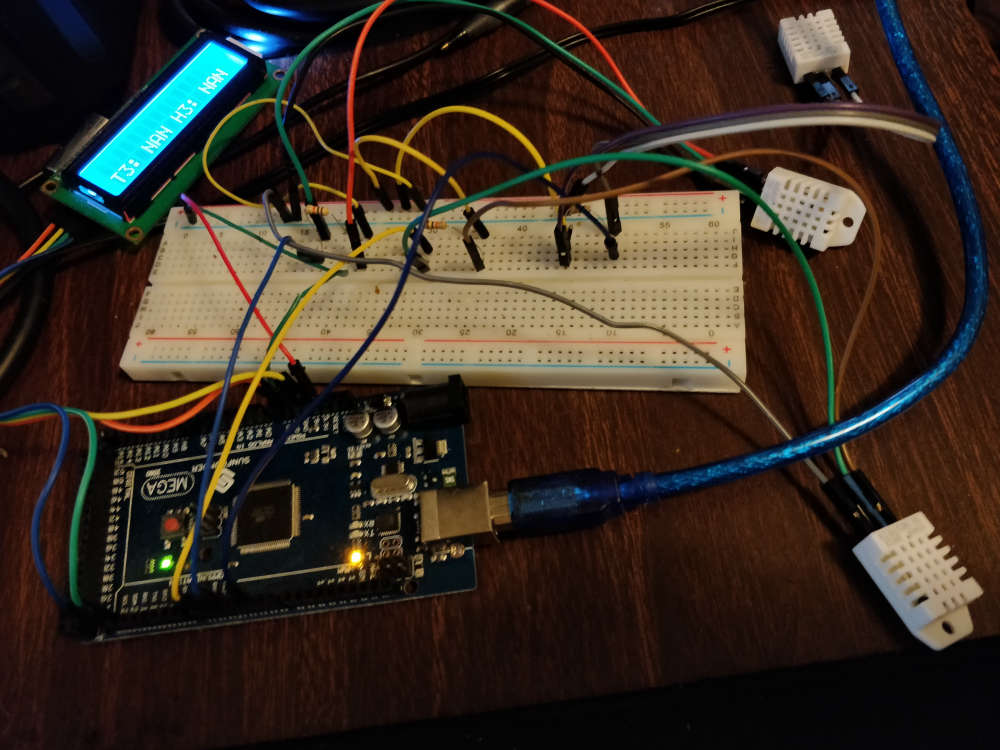3 DHT22 recieving NAN reading from Arduino ATMEGA 2560

• Hello. I'm working on a project for school. I know the code is ugly, but I need this working before I iterate it through a for loop and an array of dht objects. Right now, the first two sensors are working just fine. I'm receiving nothing but NAN on the third sensor. I have switched sensors between my wiring, and the same happens...so it's not the same sensor. I'm using the 3.3v output from the arduino (using the 5v for the lcd display) and have wired all 3 with 10k pull up resistors to the data pins. My code is IDENTICAL for sensor 3, and messing with the delays has yielded nothing. Below is my code...

#include <Wire.h>
#include <LiquidCrystal_I2C.h>
#include <DHT.h>

#define DHTPIN 2     // Digital pin connected to the DHT sensor
#define DHT2PIN 3
#define DHT3PIN 5
// Feather HUZZAH ESP8266 note: use pins 3, 4, 5, 12, 13 or 14 --
// Pin 15 can work but DHT must be disconnected during program upload.

// Uncomment whatever type you're using!
//#define DHTTYPE DHT11   // DHT 11
#define DHTTYPE DHT22   // DHT 22  (AM2302), AM2321
//#define DHTTYPE DHT21   // DHT 21 (AM2301)

// Connect pin 1 (on the left) of the sensor to +5V
// NOTE: If using a board with 3.3V logic like an Arduino Due connect pin 1
// to 3.3V instead of 5V!
// Connect pin 2 of the sensor to whatever your DHTPIN is
// Connect pin 4 (on the right) of the sensor to GROUND
// Connect a 10K resistor from pin 2 (data) to pin 1 (power) of the sensor

// Initialize DHT sensor.
// Note that older versions of this library took an optional third parameter to
// tweak the timings for faster processors.  This parameter is no longer needed
// as the current DHT reading algorithm adjusts itself to work on faster procs.

/**********************************************************/

char humid[]="      "; //the string to print on the LCD
char temp[]="      ";
char humid2[]="      "; //the string to print on the LCD
char temp2[]="      ";
char temp3[]="      ";
char humid3[]="      "; //the string to print on the LCD
int tim = 350; //the value of delay time

LiquidCrystal_I2C lcd(0x27,16,2); // set the LCD address to 0x27 for a 16 chars and 2 line display

DHT dht(2, DHT22);
DHT dht2(3, DHT22);
DHT dht3(5, DHT22);

float h = dht.readHumidity(); // Sensor readings may also be up to 2 seconds 'old' (its a very slow sensor)
float t = dht.readTemperature(); // Read temperature as Celsius (the default) // Read temperature as Fahrenheit (isFahrenheit = true)
float f = dht.readTemperature(true);

float hif = dht.computeHeatIndex(f, h);  // Compute heat index in Fahrenheit (the default)
float hic = dht.computeHeatIndex(t, h, false);  // Compute heat index in Celsius (isFahreheit = false)

float h2 = dht2.readHumidity(); // Sensor readings may also be up to 2 seconds 'old' (its a very slow sensor)
float t2 = dht2.readTemperature(); // Read temperature as Celsius (the default) // Read temperature as Fahrenheit (isFahrenheit = true)
float f2 = dht2.readTemperature(true);

float h3 = dht3.readHumidity(); // Sensor readings may also be up to 2 seconds 'old' (its a very slow sensor)
float t3 = dht3.readTemperature(); // Read temperature as Celsius (the default) // Read temperature as Fahrenheit (isFahrenheit = true)
float f3 = dht3.readTemperature(true);

void setup()
{
lcd.init(); //initialize the lcd
lcd.backlight(); //open the backlight
lcd.clear();
Serial.begin(9600);
Serial.println(F("DHTxx test!"));
//lcd.print("Test"[0,0]);
delay(tim);

dht.begin();
dht2.begin();
dht3.begin();

};

//nothing can exist... hee..r..

void loop()
{

delay(2000);
h = dht.readHumidity(); // Sensor readings may also be up to 2 seconds 'old' (its a very slow sensor)
t = dht.readTemperature(); // Read temperature as Celsius (the default) // Read temperature as Fahrenheit (isFahrenheit = true)
if (isnan(h) || isnan(t) || isnan(f)) {
Serial.println(F("Failed to read from DHT sensor 1!"));
return;    }
hif = dht.computeHeatIndex(f, h);  // Compute heat index in Fahrenheit (the default)
hic = dht.computeHeatIndex(t, h, false);  // Compute heat index in Celsius (isFahreheit = false)

h2 = dht2.readHumidity(); // Sensor readings may also be up to 2 seconds 'old' (its a very slow sensor)
t2 = dht2.readTemperature(); // Read temperature as Celsius (the default) // Read temperature as Fahrenheit (isFahrenheit = true)
if (isnan(h) || isnan(t) || isnan(f)) {
Serial.println(F("Failed to read from DHT sensor 2!"));
return;    }

delay(1000);
h3 = dht3.readHumidity(); // Sensor readings may also be up to 2 seconds 'old' (its a very slow sensor)
t3 = dht3.readTemperature(); // Read temperature as Celsius (the default) // Read temperature as Fahrenheit (isFahrenheit = true)
if (isnan(h) || isnan(t) || isnan(f)) {
Serial.println(F("Failed to read from DHT sensor 3!"));
return;    }

dtostrf(h, 2, 1, humid);
dtostrf(t, 2, 1, temp);

dtostrf(h2, 2, 1, humid2);
dtostrf(t2, 2, 1, temp2);

dtostrf(h3, 2, 1, humid3);
dtostrf(t3, 2, 1, temp3);

//t1
lcd.setCursor(0,0); // set the cursor to column 15, line 0
lcd.print("T1:");
lcd.setCursor(4,0); // set the cursor to column 15, line 0
lcd.print(temp);
//delay(1000);
//h2
lcd.setCursor(8,0); // set the cursor to column 15, line 0
lcd.print("H1:");
lcd.setCursor(12,0); // set the cursor to column 15, line 0
lcd.print(humid);
// delay(1000);

//t2
lcd.setCursor(0,1); // set the cursor to column 15, line 0
lcd.print("T2:");
lcd.setCursor(4,1); // set the cursor to column 15, line 0
lcd.print(temp2);
//delay(1000);

lcd.setCursor(8,1); // set the cursor to column 15, line 0
lcd.print("H2:");
lcd.setCursor(12,1); // set the cursor to column 15, line 0
lcd.print(humid2);
//delay(1000);

lcd.clear();
lcd.setCursor(0,1); // set the cursor to column 15, line 0
lcd.print("T3:");
lcd.setCursor(4,1); // set the cursor to column 15, line 0
lcd.print(temp3);
lcd.setCursor(8,1); // set the cursor to column 15, line 0
lcd.print("H3:");
lcd.setCursor(12,1); // set the cursor to column 15, line 0
lcd.print(humid3);

};

• @kbickham welcome to the MySensors forum.

Isn't the lcd connected to pin 5?
According to https://learn.adafruit.com/adafruit-huzzah-esp8266-breakout/pinouts pins 4 and 5 are used for i2c, and you are using an i2c lcd.

• lcd is connected to the dedicated i2c interface pins

• ![alt text]()
here are some pictures of what I have.

• I moved it to pin 6, no change. Also I realize I have the pins defined above, and then explicit feed them in to the functions.

• WOW, this crappy breadboard had discontinuities on the ground rail. switched it adjacent to the other grounds...and not it works fine!

• @kbickham thanks for clarifying. Sorry for not reading properly, I thought you used an esp8266.

• h2 = dht2.readHumidity(); // Sensor readings may also be up to 2 seconds 'old' (its a very slow sensor)
t2 = dht2.readTemperature(); // Read temperature as Celsius (the default) // Read temperature as Fahrenheit (isFahrenheit = true)
if (isnan(h) || isnan(t) || isnan(f)) {
Serial.println(F("Failed to read from DHT sensor 2!"));
return;

if (isnan(h) || isnan(t) || isnan(f)) {
should be h2, t2, f2, and the same for the third DHT• First of all, I think some of your code is wrong:
h3 = dht3.readHumidity(); // Sensor readings may also be up to 2 seconds 'old' (its a very slow sensor)
t3 = dht3.readTemperature(); // Read temperature as Celsius (the default) // Read temperature as Fahrenheit (isFahrenheit = true)
if (isnan(h) || isnan(t) || isnan(f)) {
Serial.println(F("Failed to read from DHT sensor 3!"));
return; }
It needs to be changed to if(isnan(h3) || isnan(t3) || isnan(f3)) to detect if the third sensor returns NAN.

Secondly, have you checked your wiring and the code is exactly the same as the first sensor and the second?

Third, is this situation due to insufficient power supply? I think you can check the circuit information of the DHT22.